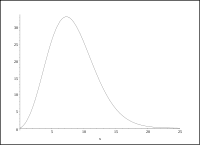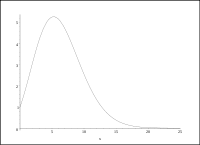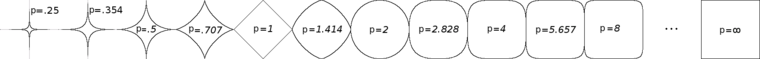# Unit sphere

﻿
Unit sphere

In mathematics, a unit sphere is the set of points of distance 1 from a fixed central point, where a generalized concept of distance may be used; a closed unit ball is the set of points of distance less than or equal to 1 from a fixed central point. Usually a specific point has been distinguished as the origin of the space under study and it is understood that a unit sphere or unit ball is centered at that point. Therefore one speaks of "the" unit ball or "the" unit sphere.

For example, a one-dimensional sphere is the surface of what is commonly called a "circle", while such a circle's interior and surface together are the two-dimensional ball. Similarly, a two-dimensional sphere is the surface of the Euclidean solid known colloquially as a "sphere", while the interior and surface together are the three-dimensional ball.

A unit sphere is simply a sphere of radius one. The importance of the unit sphere is that any sphere can be transformed to a unit sphere by a combination of translation and scaling. In this way the properties of spheres in general can be reduced to the study of the unit sphere.

## Unit spheres and balls in Euclidean space

In Euclidean space of n dimensions, the unit sphere is the set of all points$(x_1, \ldots, x_n)$ which satisfy the equation$x_1^2 + x_2^2 + \cdots + x_n ^2 = 1$

and the closed unit ball is the set of all points satisfying the inequality$x_1^2 + x_2^2 + \cdots + x_n ^2 \le 1.$

### General area and volume formulas

The classical equation of a unit sphere is that of the ellipsoid with a radius of 1 and no alterations to the x-, y-, or z- axes:

f(x,y,z) = x2 + y2 + z2 = 1

The volume of the unit ball in n-dimensional Euclidean space, and the surface area of the unit sphere, appear in many important formulas of analysis. The volume of the unit ball in n dimensions, which we denote Vn, can be expressed by making use of the Gamma function. It is$V_n = \frac{\pi ^ {n/2}}{\Gamma(1+n/2)} = \begin{cases} {\pi^{n/2}}/{(n/2)!} & \mathrm{if~}n \ge 0\mathrm{~is~even,} \\ ~\\ {\pi^{\lfloor n/2 \rfloor}2^{\lceil n/2 \rceil}}/{n!!} & \mathrm{if~}n \ge 0\mathrm{~is~odd,} \end{cases}$

where n!! is the double factorial.

The hypervolume of the (n–1)-dimensional unit sphere (i.e., the "area" of the surface of the n-dimensional unit ball), which we denote An, can be expressed as$A_n = n V_n = \frac{n \pi ^ {n/2}}{\Gamma(1+n/2)} = \frac{2 \pi ^ {n/2}}{\Gamma(n/2)}\,,$

where the last equality holds only for n > 0.

The surface areas and the volumes for some values of n are as follows:

n An (surface area) Vn (volume)
0 0(1 / 0!)π0 0.000 (1 / 0!)π0 1.000
1 1(21 / 1!!)π0 2.000 (21 / 1!!)π0 2.000
2 2(1 / 1!)π1 = 2π 6.283 (1 / 1!)π1 = π 3.142
3 3(22 / 3!!)π1 = 4π 12.57 (22 / 3!!)π1 = (4 / 3)π 4.189
4 4(1 / 2!)π2 = 2π2 19.74 (1 / 2!)π2 = (1 / 2)π2 4.935
5 5(23 / 5!!)π2 = (8 / 3)π2 26.32 (23 / 5!!)π2 = (8 / 15)π2 5.264
6 6(1 / 3!)π3 = π3 31.01 (1 / 3!)π3 = (1 / 6)π3 5.168
7 7(24 / 7!!)π3 = (16 / 15)π3 33.07 (24 / 7!!)π3 = (16 / 105)π3 4.725
8 8(1 / 4!)π4 = (1 / 3)π4 32.47 (1 / 4!)π4 = (1 / 24)π4 4.059
9 9(25 / 9!!)π4 = (32 / 105)π4 29.69 (25 / 9!!)π4 = (32 / 945)π4 3.299
10 10(1 / 5!)π5 = (1 / 12)π5 25.50 (1 / 5!)π5 = (1 / 120)π5 2.550

where the decimal expanded values for n ≥ 2 are rounded to the displayed precision.

#### Recursion

The An values satisfy the recursion:

A0 = 0
A1 = 2
A2 = 2π$A_n = \frac{2 \pi}{n-2} A_{n-2}$ for n > 2.

The Vn values satisfy the recursion:

V0 = 1
V1 = 2$V_n = \frac{2 \pi}{n} V_{n-2}$ for n > 1.

#### Fractional dimensions

The formulae for An and Vn can be computed for any real number n ≥ 0, and there are circumstances under which it is appropriate to seek the sphere area or ball volume when n is not a non-negative integer.This shows the hypervolume of an (x–1)-dimensional sphere (i.e., the "area" of the surface of the x-dimensional unit ball) as a continuous function of x.This shows the volume of a ball in x dimensions as a continuous function of x.

The surface area of an (n–1)-dimensional sphere with radius r is An rn−1 and the volume of an n-dimensional ball with radius r is Vn rn. For instance, the area is A = 4πr 2 for the surface of the three-dimensional ball of radius r. The volume is V = 4πr 3 / 3 for the three-dimensional ball of radius r.

## Unit balls in normed vector spaces

More precisely, the open unit ball in a normed vector space V, with the norm$\|\cdot\|$, is$\{ x\in V: \|x\|<1 \}.$

It is the interior of the closed unit ball of (V,||·||),$\{ x\in V: \|x\|\le 1\}.$

The latter is the disjoint union of the former and their common border, the unit sphere of (V,||·||),$\{ x\in V: \|x\| = 1 \}.$

The 'shape' of the unit ball is entirely dependent on the chosen norm; it may well have 'corners', and for example may look like [−1,1]n, in the case of the norm l in Rn. The round ball is understood as the usual Hilbert space norm, based in the finite dimensional case on the Euclidean distance; its boundary is what is usually meant by the unit sphere. Here are some images of the unit ball for the two-dimensional$\ell^p$ space for various values of p (the unit ball being concave for p < 1 and convex for p ≥ 1):These illustrate why the condition p ≥ 1 is necessary in the definition of the$\ell^p$ norm, as the unit ball in any normed space must be convex as a consequence of the triangle inequality.

Note that for the circumferences Cp of the two-dimensional unit balls we have:$C_{0} = C_{\infty} = 8$ is the maximum value.$C_{1} = 4 \sqrt{2}$ is the minimum value.$C_{2} = 2 \pi \,.$

## Generalizations

### Metric spaces

All three of the above definitions can be straightforwardly generalized to a metric space, with respect to a chosen origin. However, topological considerations (interior, closure, border) need not apply in the same way (e.g., in ultrametric spaces, all of the three are simultaneously open and closed sets), and the unit sphere may even be empty in some metric spaces.

If V is a linear space with a real quadratic form F:V → R, then { p ∈ V : F(p) = 1 } is sometimes called the unit quasi-sphere of V. For example, the quadratic form x2y2 corresponds to a unit quasi-sphere that is a hyperbola which plays the role of the "unit circle" in the plane of split-complex numbers. Similarly, quadratic form x2 yields a pair of lines for the unit quasi-sphere in the dual number plane.

Wikimedia Foundation. 2010.

### Look at other dictionaries:

• unit sphere — unit circle or unit sphere, Mathematics. a circle or sphere whose radius is one unit of distance …   Useful english dictionary

• Contractibility of unit sphere in Hilbert space — In topology, it is a surprising fact that the unit sphere in (infinite dimensional) Hilbert space is a contractible space, sinceno finite dimensional spheres are contractible.This can be demonstrated in several different ways. Topological proof… …   Wikipedia

• Unit disk — For other uses, see Disc (disambiguation). An open Euclidean unit disk In mathematics, the open unit disk (or disc) around P (where P is a given point in the plane), is the set of points whose distance from P is less than 1 …   Wikipedia

• Unit tangent bundle — In mathematics, the unit tangent bundle of a Finsler manifold ( M , || . ||), denoted by UT( M ) or simply UT M , is a fiber bundle over M given by the disjoint union:mathrm{UT} (M) := coprod {x in M} left{ v in mathrm{T} {x} (M) left| | v | {x} …   Wikipedia

• Sphere — Globose redirects here. See also Globose nucleus. A sphere (from Greek σφαίρα sphaira , globe, ball, [ [http://www.perseus.tufts.edu/cgi bin/ptext?doc=Perseus%3Atext%3A1999.04.0057%3Aentry%3D%23101561 Sphaira, Henry George Liddell, Robert Scott,… …   Wikipedia

• unit circle — noun : a circle whose radius is one unit of length long * * * Math. a circle whose radius has a length of one unit. [1950 55] * * * unit circle or unit sphere, Mathematics. a circle or sphere whose radius is one unit of distance …   Useful english dictionary

• Unit circle — In mathematics, a unit circle is a circle with a unit radius, i.e., a circle whose radius is 1. Frequently, especially in trigonometry, the unit circle is the circle of radius 1 centered at the origin (0, 0) in the Cartesian coordinate system in… …   Wikipedia

• Unit cube — A unit cube is a cube all of whose sides are 1 unit long. The volume of a 3 dimensional unit cube is 1 cubic unit, and its total surface area is 6 square units. Unit Hypercube The term unit cube or unit hypercube is also used to refer to… …   Wikipedia

• Unit square — The unit square is a square with all of the side lengths equalling 1.In the real planeIn a Cartesian coordinate system with coordinates ( x , y ) the unit square is defined as the square consisting of the points where both x and y lie in the unit …   Wikipedia

• Unit 731 — The Unit 731 complex Location Pingfang Coordinates …   Wikipedia